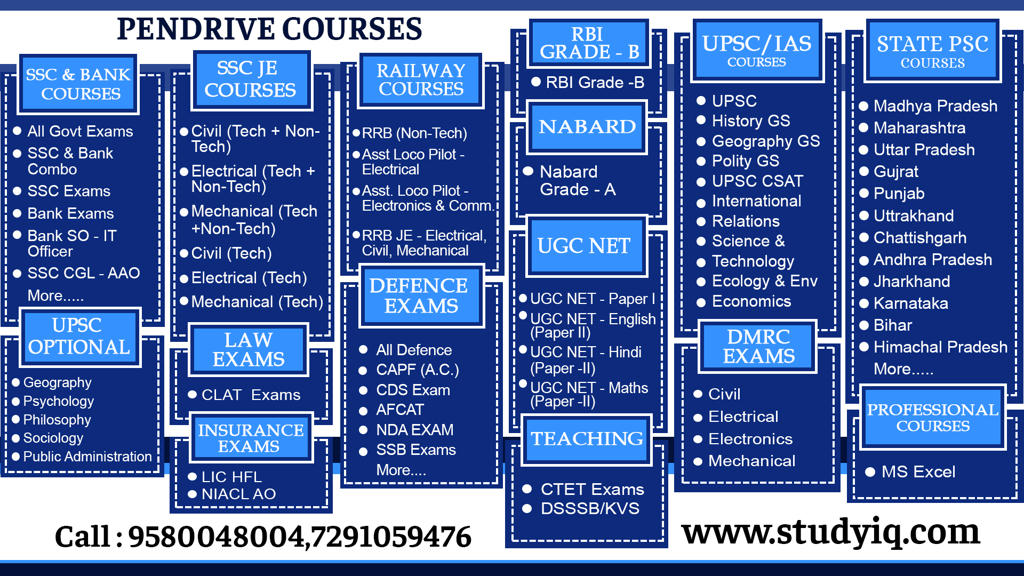# Average Questions – Part 3 – Maths & Reasoning | PDF DownloadQ1. The average age of 7 students is 18 years. If one more student comes in, then the average become 19, according to this what the new number added in this

1) 28

2) 26

3) 27

4) 30

Q2. The average age of 14 students and one of their teachers is 15 years. If the age of the teacher is removed then the average decreases by 1 year. What is the age of the teacher?

1) 29y

2) 35y

3) 32y

4) 30y

Q3. The average of 5 numbers is 20, if one number is removed, the average is 15. So which number is removed?

1) 5

2) 35

3) 112

4) 45

Q4. Average of 10 number is 20. If 5 is subtracted from each number. Find a new average?

Q5. Average of 20 numbers is 25. If each number is multiplied by 2. Find the new average?

Q6. The average temperature from Monday to Wednesday is 37 degrees. Whereas the average temperature from Tuesday to Thursday is 34 degrees. Temperature of Thursday is 4/5 of temperature of Monday. Find the temperature of Thursday.

Q7. The average temperature of Monday, Tuesday, Wednesday, Thursday is 31 degree. And average temperature of Tuesday, Wednesday, Thursday and Friday is 29.5 degree. Average temperature of Monday is 1 37 2 % more than temperature of Friday. Find temperature of Monday?

Q8. Average weight of A,B,C is 84 Kg. If D is also included their average weight become 80 Kg. If another man E whose weight is 3 Kg more than D came in place of A then average weight of B,C,D,E will become 79 Kg. Find weight of A?

Q9. Sachin Tendulkar has a certain average of 11 innings; he scored 120 runs in his 12th innings and increased the average by 5 runs, so how much is new average?

1) 60

2) 62

3) 65

4) 66

Q10. A batsman scored runs in 11 innings at a certain average. That batsman scored 90 runs in the 12th innings. Whereby his Average 5 runs decreased. Accordingly, how much is the average of that batsman after 12 innings.

1) 127

2) 145

3) 150

4) 140

Q11. The average of 10 numbers is 30. Later it is found that two of those numbers were written incorrectly at 51, 32 instead of 15, 23. What is the correct average accordingly?

1) 25.5

2) 32

3) 30

4) 34.5

Q12. The average value of 20 observations is 75. Later, it was found that 97 of them has read 79, so was the correct average value?

1) 75.7

2) 75.8

3) 75.9

4) 75.6

Q13. Average score of 60 students was found in an examination. By removing qualitative errors, the average score of some 100 test takers was 30 instead 60 and the average of all the examiners reduced to 45 points. According to the number of candidates who participated in that examination who participated?

1) 200

2) 210

3) 240

4) 180